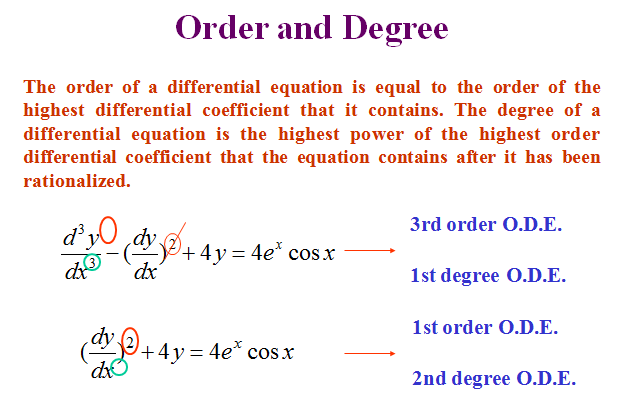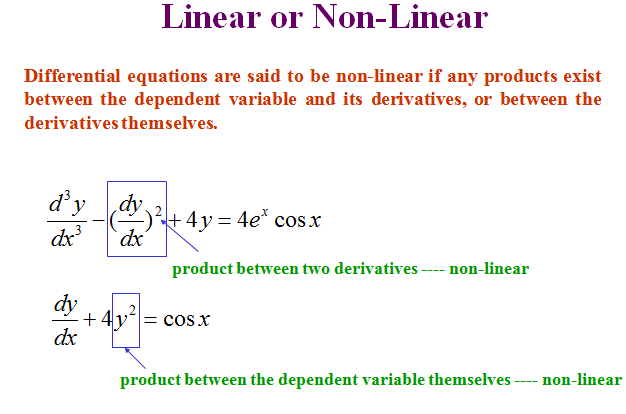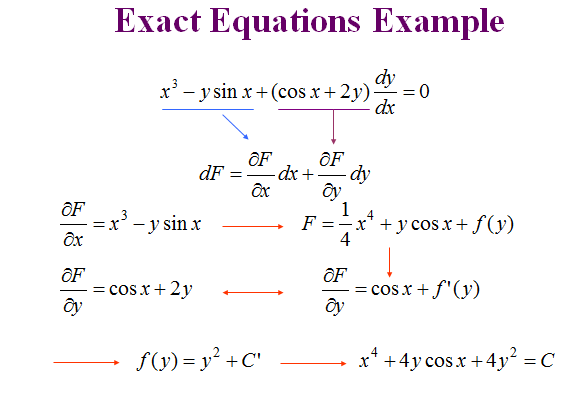# SCC Education

## Solving Models Related to Ordinary Differential Equations

Solving Models Related to Ordinary Differential Equations
Differential Equation
An equation relating a dependent variable to one or more independent variables by means of its differential coefficients with respect to the independent variables is called a “differential equation”.some-important-formulas-of-integration

solving-models-related-to-ordinary differential equation

differentiation-of-exponential-functions

area-between-curve

vector

application-of-derivatives

homogenous-differential-equation

inverse-trigonometry-questions

integration

integration-questions

Rolles-theorem-and-mean-value-theorem

Techniques-of-integration-substitution

critical-points-and-point-of-inflection

intermediate-value-theorem

### Sample paper Mathematics 12